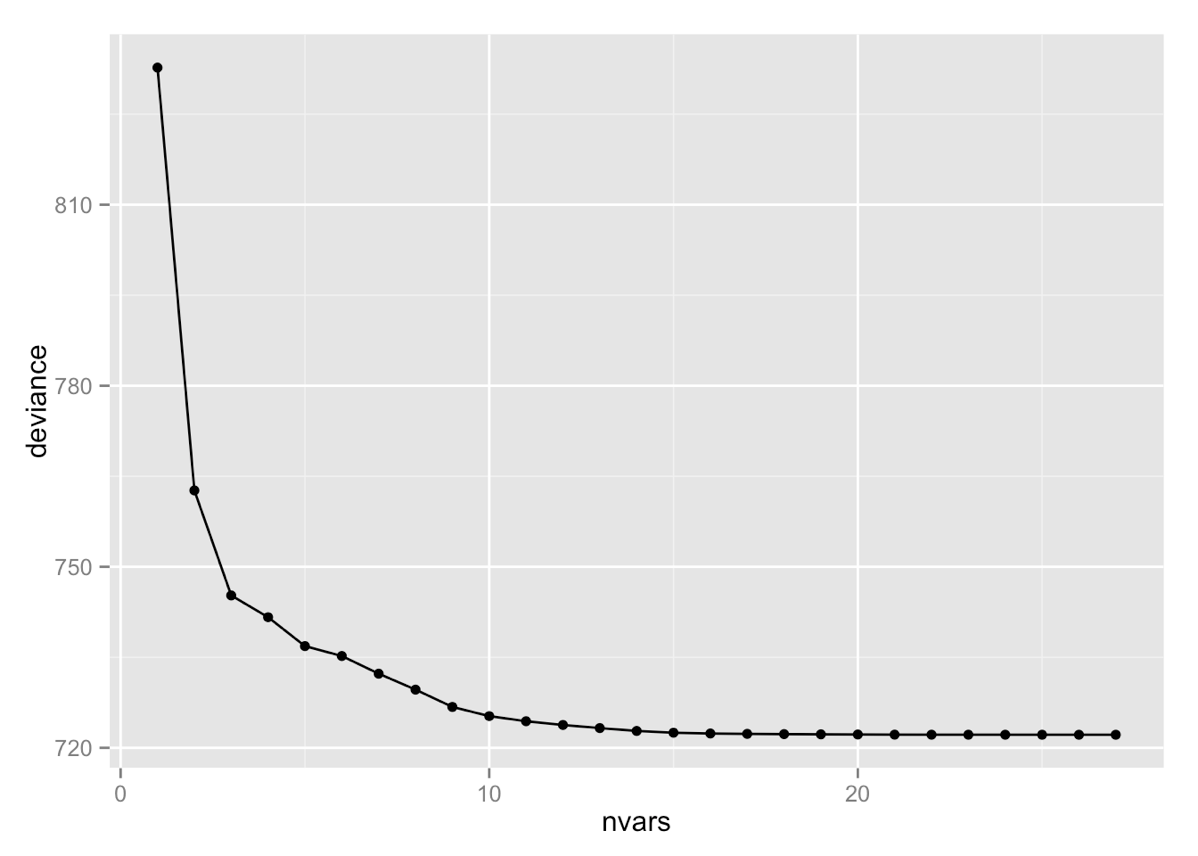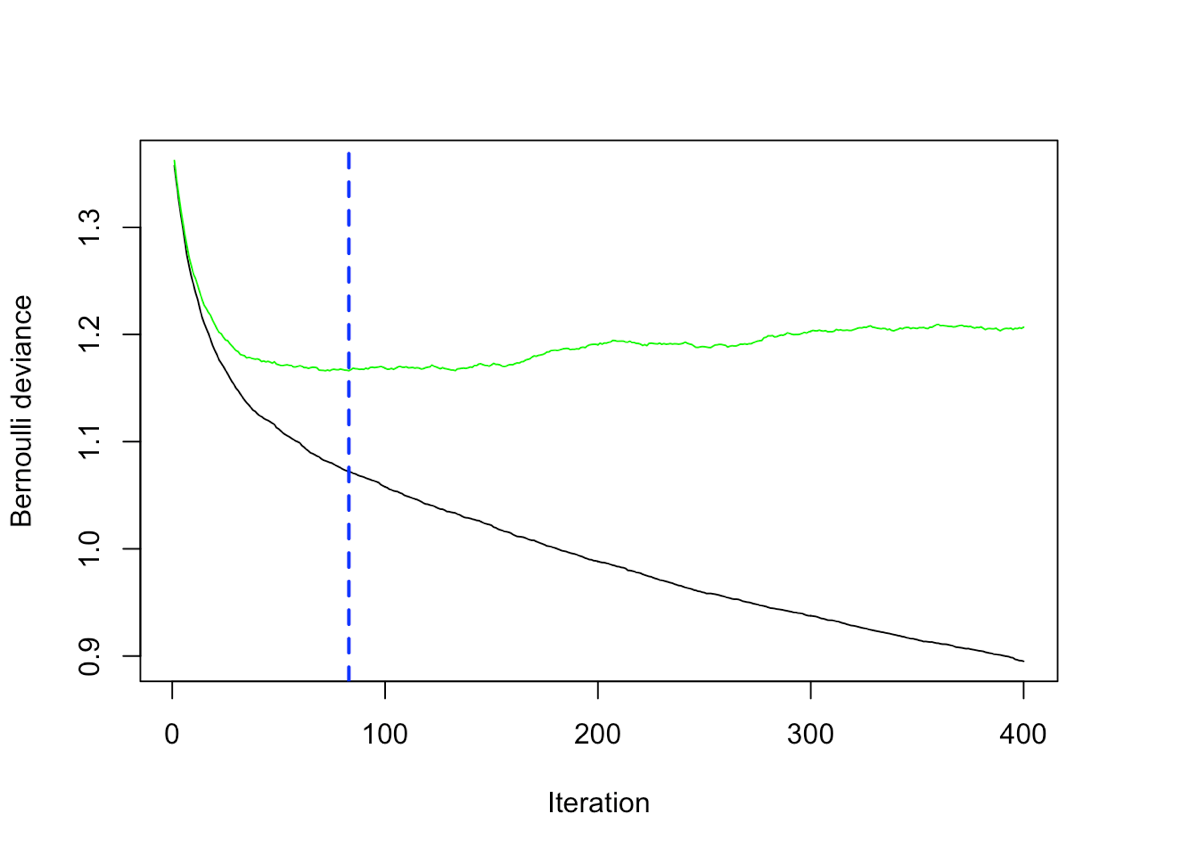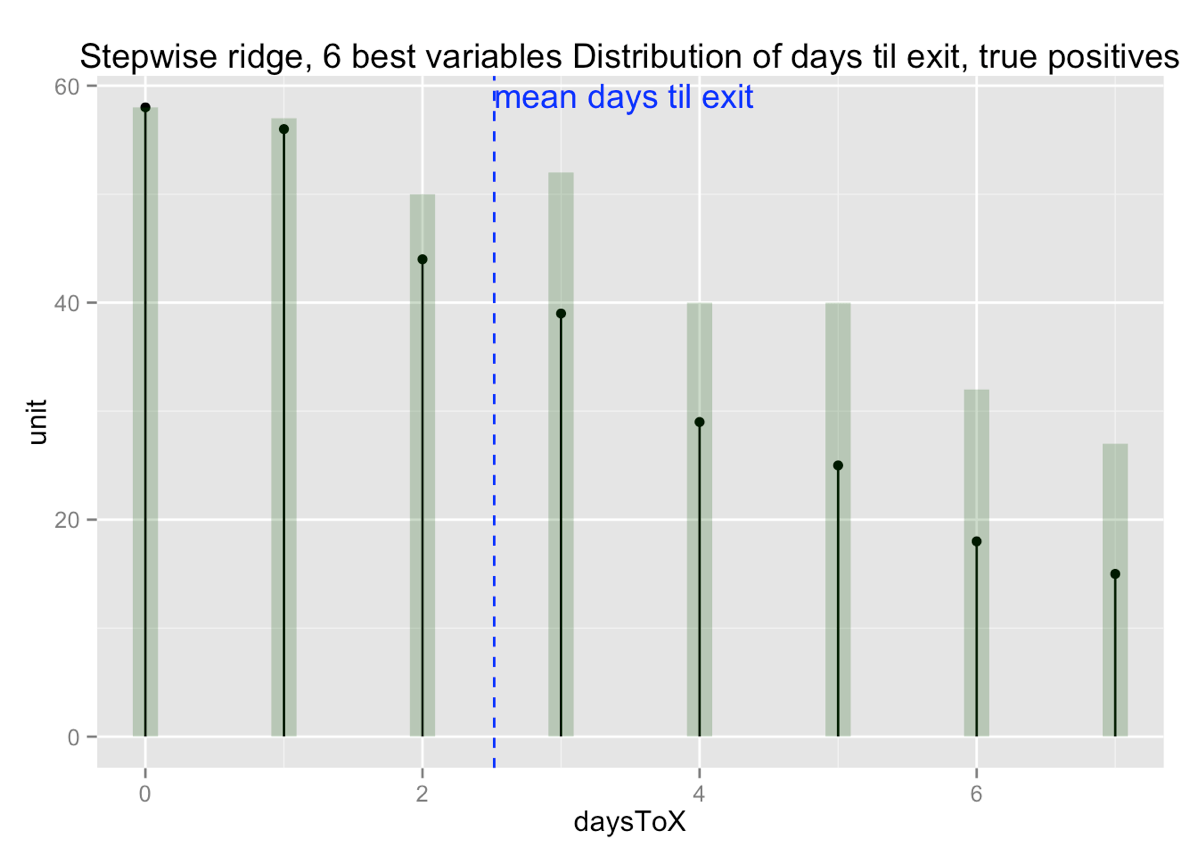# Working with Sessionized Data 2: Variable Selection

In our previous post in this series, we introduced sessionization, or converting log data into a form that’s suitable for analysis. We looked at basic considerations, like dealing with time, choosing an appropriate dataset for training models, and choosing appropriate (and achievable) business goals. In that previous example, we sessionized the data by considering all possible aggregations (window widths) of the data as features. Such naive sessionization can quickly lead to very wide data sets, with potentially more features than you have datums (and collinear features, as well). In this post, we will use the same example, but try to select our features more intelligently.Illustration: Boris Artzybasheff
photo: James Vaughan, some rights reserved

The Example Problem

Recall that you have a mobile app with both free (A) and paid (B) actions; if a customer’s tasks involve too many paid actions, they will abandon the app. Your goal is to detect when a customer is in a state when they are likely to abandon, and offer them (perhaps through an in-app ad) a more economical alternative, for example a “Pro User” subscription that allows them to do what they are currently doing at a lower rate. You don’t want to be too aggressive about showing customers this ad, because showing it to someone who doesn’t need the subscription service is likely to antagonize them (and convince them to stop using your app).

You want to build a model that predicts whether a customer will abandon the app (“exit”) within seven days. Your training set is a set of 648 customers who were present on a specific reference day (“day 0”); their activity on day 0 and the ten days previous to that (days 1 through 10), and how many days until each customer exited (`Inf` for customers who never exit), counting from day 0. For each day, you constructed all possible windows within those ten days, and counted the relative rates of A events and B events in each window. This gives you 132 features per row. You also have a hold-out set of 660 customers, with the same structure. You can download the wide data set used for these examples as an `.rData` file here. The explanation of the variable names is in the previous post in this series.

In the previous installment, we built a regularized (ridge) logistic regression model over all 132 features. This model didn’t perform too badly, but in general there is more danger of overfitting when working with very wide data sets; in addition, it is quite expensive to analyze a large number of variables with standard implementations of logistic regression. In this installment, we will look for potentially more robust and less expensive ways of analyzing this data.

The Ideal Case

Ideally you would know some appropriate window lengths, from understanding of the domain. For instance, if you knew that the trend towards abandonment manifested itself over the course of a month, then weekly or twice-a-week aggregations might be all you need. But perhaps you aren’t entirely sure what the appropriate aggregation windows are. Is there any way of teasing them out?

Greedy Forward Stepwise Regression

One way to find the best features is to pick them one at a time: find the one-variable model that optimizes some model quality function, then add another variable that, combined with the first, again optimizes model quality, and so on, until the model “stops improving.” I’ve put that in quotes because in general, one stops when the incremental improvement is smaller than some threshold. Because many standard model quality metrics, like R-squared, squared error, or deviance, tend to improve as the number of parameters increases (potentially leading to bias and overfit), standard stepwise regression uses criteria like the AIC or BIC, which attempt to compensate for the complexity of the model. Here (for pedagogical purposes) we will step by hand rather than use R’s `step()` function, and will simply minimize deviance, and use an ad-hoc procedure for picking an appropriate number of variables.

As before, we’ll use L2-regularized logistic regression as the base model.

```library(glmnet)

# stepwise ridge regression: add one more variable
# to existing model
#
# xframe: data frame of independent variables
# y: vector of dependent variable
# current_vars: variables in the current model
# current_dev: deviance of current model
# candidate_vars: variables to be potentially added
#                 to model
# Returns:
#  new set of current_vars
#  new current_dev
#  improvement from previous model
add_var = function(xframe, y, current_vars, current_dev,
candidate_vars) {
best_dev = current_dev
newvar = NULL
for(var in candidate_vars) {
active=c(current_vars, var)
xf = xframe[,active]
if(length(active) > 1) {
model = glmnet(as.matrix(xf), y,
alpha=0, lambda=0.001,
family="binomial")
} else {
# glmnet requires > 1 variable
model =glm.fit(xframe[,active], y,
}
moddev = deviance(model)
if(moddev < best_dev) {
newvar = var
best_dev = moddev
}
}
improvement = 1 - (best_dev/current_dev)
list(current_vars= c(current_vars, newvar),
current_dev = best_dev,
improvement = improvement)
}
# stepwise ridge regression: entire loop
#
# data: training data frame
# vars: variables to consider
# yVar: name of dependent variable
# min_improve: terminate when model
#              improvement is less than this
#
# returns final set of variables,
# along with improvements and deviances
stepwise_ridge = function(data, vars, yVar,
min_improve=1e-6) {
current_vars=c()
candidate_vars = vars
devs = numeric(length(vars))
improvement = numeric(length(vars))
current_dev=null_deviance(data[[yVar]])
do_continue=TRUE
while(do_continue) {
current_dev, candidate_vars)
current_vars = iter\$current_vars
current_dev = iter\$current_dev
count = length(current_vars)
devs[count] = current_dev
improvement[count] = iter\$improvement
candidate_vars = setdiff(vars, current_vars)
do_continue =
(length(candidate_vars) > 0) &&
(iter\$improvement > min_improve)
}
list(current_vars = current_vars,
deviances=devs,
improvement=improvement)

# load vars (names of vars), yVar (name of y column),
# dTrainS, dTestS

# number of candidate variables
length(vars)
##  132

# fix the Infs in the training data
# shouldn't be many of them
isInf = dTrainS\$daysToX == Inf
maxfinite = max(dTrainS\$daysToX[!isInf])
dTrainS\$daysToX[isInf] = maxfinite

# null deviance:
# the deviance of the mean value
# of the y variable
null_deviance(dTrainS[[yVar]])
##  892.3776
# model using all variables
allvar_model = ridge_model(dTrainS[,vars],
dTrainS[[yVar]])

# the deviance of the model
# with all variables
deviance(allvar_model)
##  722.1471

# greedy forward stepwise regression
modelparams = stepwise_ridge(dTrainS, vars, yVar)
current_vars = modelparams\$current_vars
devs = modelparams\$deviances
improvement=modelparams\$improvement

# number of variables selected
length(current_vars)
##  27

final_model = ridge_model(dTrainS[,current_vars],
dTrainS[[yVar]])
final_model\$deviance
##  722.1666

current_vars[1:7]
## "B_1_1"   "A_0_0"   "B_6_0"
## "B_7_2"   "B_9_3"   "A_1_0"   "A_5_5"

}

```

We can reduce the number of variables from 132 to 27 without substantially increasing the training deviance (recall that large deviance is bad).

If we look at the first few selected variables, we see that the model looks at the rate of B events occurring “yesterday” (`B_1_1`) and compares it with the rate of B events over sliding windows of 6-7 days from today, yesterday, and the day before yesterday. It also looks at the rate of A events from today and yesterday (and 5 days ago). Recall that in this simulated data a customer’s rates of A and B actions stay constant until they switch to the “at-risk” state, at which time their rate of B actions increases to a new constant (see the previous installment) — in other words, there is an edge after which the customer’s B rate is notably higher. Given that knowledge (which we of course wouldn’t have in a real data situation), comparing the current B rate with running averages from the last few days makes sense.

So by simply stepping through the variables that we generated through naive sessionization, we can reduce the number of features to a more tractable number. If fact, we suspect that we can decrease the number of variables even more. Let’s look at how deviance changed as we added variables.The top plot is deviance as a function of the number of variables, the bottom plot is the improvement from the previous model — kind of the “derivative of the deviance.” After about ten variables, the model improvement leveled off. It’s a folk theorem, when looking at graphs like these (model quality as a function of a parameter) that the optimal value for the parameter occurs at the “elbow” of the model quality graph, or alternatively at either the maximum or elbow of the improvement graph. Which point is the elbow of this deviance graph is a fuzzy question; the improvement graph is easier to read. The maximum is 2 variables; the elbow is 4. There’s an argument to be made for 6 variables, too, so let’s look at all these models, this time on hold-out data.

```# more reduced models
final2_model = ridge_model(dTrainS[,current_vars[1:2]], dTrainS[[yVar]])
final4_model = ridge_model(dTrainS[,current_vars[1:4]], dTrainS[[yVar]])
final6_model = ridge_model(dTrainS[,current_vars[1:6]], dTrainS[[yVar]])

# Compare all the (non-trivial) models on holdout data
# See https://github.com/WinVector/SessionExample/blob/master/NarrowChurnModel.Rmd
# for the evaluate() function code

rbind(evaluate(allvar_model, dTestS, dTestS[[yVar]], "all variables"),
evaluate(final_model, dTestS, dTestS[[yVar]], "stepwise run"),
evaluate(final2_model, dTestS, dTestS[[yVar]], "best 2 variables"),
evaluate(final4_model, dTestS, dTestS[[yVar]], "best 4 variables"),
evaluate(final6_model, dTestS, dTestS[[yVar]], "best 6 variables"))

##              label deviance    recall precision  accuracy
## 1    all variables 756.6919 0.7752809 0.7360000 0.7287879
## 2     stepwise run 755.8788 0.7752809 0.7360000 0.7287879
## 3 best 2 variables 769.7035 0.7696629 0.7080103 0.7045455
## 4 best 4 variables 743.1230 0.7921348 0.7540107 0.7484848
## 5 best 6 variables 743.2160 0.7977528 0.7533156 0.7500000
```

The four-variable model dominates all the others on the hold-out data on deviance and precision, and isn’t too far behind the six-variable model on recall and accuracy. This indicates that the model using all the variables was slightly overfitting, as was even the model with 27 variables. For domain reasons, you still might prefer to use the six-variable model — I would feel more comfortable using 3 running average measurements instead of two, and I like having more A rate information in the model. The performance difference between the two models is slight, and 6 variables is still far, far fewer than 132.

Note that we could also have used n-fold cross validation to select the best number of variables.

Discussion

This approach isn’t perfect. You still have to generate all the naive sessionization features, and you still have to run through them all, multiple times. However, if M is the number of naive sessionization features, and M is large, then fitting M*k small logistic regression models (where k < M) may still be less expensive than fitting one logistic regression model of size M. Also, if M is so large that you have trouble fitting it in memory (it can happen), you can simply generate each feature on the fly, as needed.

If you really want to, you can cut down the computation a little by not fitting a model to all the current variables at every step; you can freeze the previous model and use its predictions as an offset to the next model (via the `offset` parameter). This means you are only fitting a single-variable model at every iteration. If you do this, it’s a good idea to do one last polishing step at the end by refitting all the selected variables at once.

You can interpret freezing the previous model and using it as an offset to the next model as minimizing the “residual deviance” at every iteration. If this sounds familiar, it should: incrementally building up a model by minimizing residual deviance and iterating is one of the basic ideas behind gradient boosting, though the details are different, and gradient boosting usually boosts trees rather than single-variable models. So rather than trying the procedure I just described, why don’t we just try gradient boosting?

We’ll use the `gbm()` function from the `gbm` package, with `interaction.depth=1`, since we didn’t use interactions in our logistic regression models.

```library(gbm)

# wrapper functions for prediction
gbm_predict_function = function(model, nTrees) {
force(model)
function(xframe) {
predict(model,newdata=xframe,type='response',
n.trees=nTrees)
}
}

# wrapper function for fitting.
# Returns: a prediction function
#          variable influences
gbm_model = function(dframe, formula, weights=NULL) {
if(is.null(weights)) {
nrows = dim(dframe)
weights=numeric(nrows)+1 # all 1
}
modelGBM```

The function `gbm.perf()` (as we’ve called it in `gbm_model()`) uses cross-validation to pick the optimal number of boosting iterations (trees):The black curve shows model deviance on training data as a function of the number of iterations; the green curve shows model deviance on holdout. The algorithm selects the point where the holdout deviance begins to increase again: in this case, 83 trees. Since we have set the interaction depth to 1, this is essentially the number of variables in the model.

We can compare the resulting model to our (reduced) stepwise model.

```# compare to the best ridge model
bestridge_model = final6_model
bestn = 6

rbind(evaluate(bestridge_model, dTestS, dTestS[[yVar]],
"best stepwise model"),
evaluate(modelGBM, dTestS, dTestS[[yVar]],
"gbm model, interaction=1"))

##                      label deviance    recall precision  accuracy
## 1      best stepwise model 743.2160 0.7977528 0.7533156 0.7500000
## 2 gbm model, interaction=1 728.4487 0.7387640 0.7758112 0.7439394
```

The gradient boosting model has lower deviance on hold-out, so it’s predicting probabilities better. It’s also more precise, but has lower recall. Unfortunately, if you want to use the gbm model, you still have to use all the features as input, so you lose the variable reduction advantage, not only during model application, but during model fitting — this matters if you can’t get all the features into memory at once.

The summary of a `gbm` model returns the variable influences, which we can use as proxies for variable importance. So you can try the “elbow” trick on the graph of influence versus number of variables, then refit a model using only those variables. I won’t show the graph here, but I decided on 7 variables, not only because it appeared to be an elbow on the influence graph, but also because 7 is nearly the same number of variables as we used in our reduced logistic regression model. The resulting variables are different from those the stepwise procedure selected:

```##         var   rel.inf
## B_3_0 B_3_0 25.610258
## B_2_0 B_2_0 16.911399
## B_4_0 B_4_0 14.006369
## A_2_0 A_2_0 12.537114
## A_0_0 A_0_0 11.648087
## A_1_0 A_1_0 11.206434
## B_1_0 B_1_0  8.080339
```

The performance of the reduced gbm model is similar to that of the full model, and also similar to the performance of the reduced logistic regression model. Again the gradient boosting models have better deviance, but inferior recall.

```##                               label deviance    recall precision
##                 best stepwise model 743.2160 0.7977528 0.7533156
##            gbm model, interaction=1 728.4487 0.7387640 0.7758112
##   gbm model with best gbm variables 720.9506 0.7303371 0.7784431
##  accuracy
## 0.7500000
## 0.7439394
## 0.7424242
```

Recall that in addition to accurate classification, you want the model to identify about-to-exit customers early enough for you to intervene with them. So you also want to compare the reduced stepwise and gradient boosted models for the timeliness of their predictions. Here, we show the distribution of days to exit for all customers who exited within 7 days in the hold out set (shown as the green bars), along with how many of those customers each model identified (shown as the points with stems).Both models did a good job identifying customers who will exit “today” or “tomorrow” (perhaps too soon for you to intervene with them), but the stepwise regression model did a little better at early identification of customers who will exit in three to seven days.

Takeaways

• Though it is not a complete substitute for domain knowledge, stepwise regression can be a useful tool for teasing out good predictive variables from a very large pool of candidate variables in sessionized data — or in any very wide dataset.
• Since additional variables can artificially improve the training performance of a model, hold-out evaluation is essential when evaluating a model found by stepwise procedures. Either use hold-out data as we did in this post, or use cross-validation measurements like the PRESS statistic.
• Stepwise regression and gradient boosting are fairly related ideas.

You can download the wide sessionized data sets that we used in this post here.

You can download an R markdown script showing all the steps we did in this post (and more) here.

Next:

We will continue to explain important steps in sessionization.### nzumel

Data scientist with Win Vector LLC. I also dance, read ghost stories and folklore, and sometimes blog about it all.